# Unknown resistor in a series-parallel circuit

• Engineering
• flexj624
In summary, the author is trying to solve a problem but does not know how to begin. He is looking for the equivalent resistance of the circuit and then calculating current and voltage drop across each resistor. If he had the value of resistor R3 he could do this, but because he does not have it he cannot. He asks for help and hints from people on the forum.

#### flexj624

Hey guys, I have tried and tried to find a start to this problem with no success. My book may have the answer but unfortunately I must be not realizing it. So hopefully I just need a different perspective. Typically I would find the equivalent resistance of the circuit and then begin to calculate current and voltage drop across each resistor. In this situation I would find the resistance of the parallel resistors R2 and R3 by using the reciprocal of the sum of the reciprocals of the resistors equation, then add them to the series resistor R1. But because I do not have the value of R3 I can't do that. And because I don't have the current in the circuit I can't find the voltage drops to work my way around the circuit. Does anyone know how to begin this problem? Any hints would be greatly appreciated.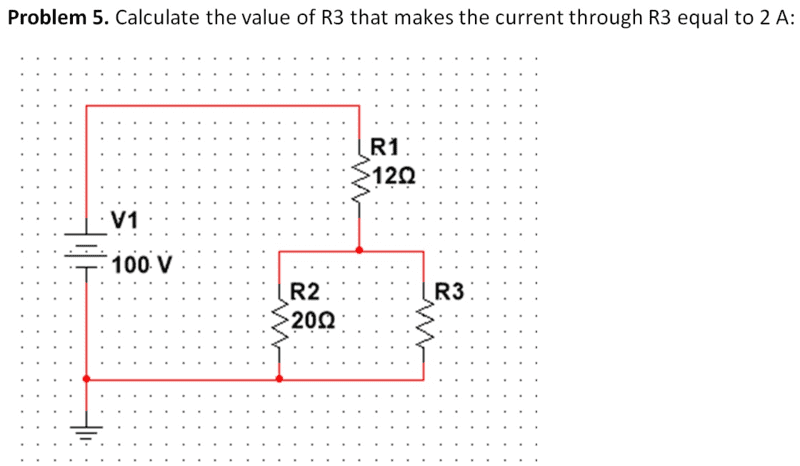## Homework Equations

Ohm's Law, resistance in parallel circuits, and resistance in series circuits.

## The Attempt at a Solution

I don't know where to start on this one because my typical starting point is finding the equivalent resistance.

#### Attachments

flexj624 said:
Hey guys, I have tried and tried to find a start to this problem with no success. My book may have the answer but unfortunately I must be not realizing it. So hopefully I just need a different perspective. Typically I would find the equivalent resistance of the circuit and then begin to calculate current and voltage drop across each resistor. In this situation I would find the resistance of the parallel resistors R2 and R3 by using the reciprocal of the sum of the reciprocals of the resistors equation, then add them to the series resistor R1. But because I do not have the value of R3 I can't do that. And because I don't have the current in the circuit I can't find the voltage drops to work my way around the circuit. Does anyone know how to begin this problem? Any hints would be greatly appreciated.
View attachment 226524

## Homework Equations

Ohm's Law, resistance in parallel circuits, and resistance in series circuits.

## The Attempt at a Solution

I don't know where to start on this one because my typical starting point is finding the equivalent resistance.

There are 3 unknowns: ##R_3##, ##I_1##, ##I_2##, where ##I_1## is the current flowing through resistor ##R_1##, ##I_2## is the current flowing through resistor ##R_2##. You are given that you want ##I_3 = 2A## (the flow through resister ##R_3##). So if you can come up with 3 equations relating those quantities to the known quantities ##I_3##, ##R_1##, ##R_2##, and ##V_1##, then you can solve the problem.

Hint: Apply nodal analysis where the resistors all come together.

•cnh1995
flexj624 said:
Hey guys, I have tried and tried to find a start to this problem with no success. My book may have the answer but unfortunately I must be not realizing it. So hopefully I just need a different perspective. Typically I would find the equivalent resistance of the circuit and then begin to calculate current and voltage drop across each resistor. In this situation I would find the resistance of the parallel resistors R2 and R3 by using the reciprocal of the sum of the reciprocals of the resistors equation, then add them to the series resistor R1. But because I do not have the value of R3 I can't do that. And because I don't have the current in the circuit I can't find the voltage drops to work my way around the circuit. Does anyone know how to begin this problem? Any hints would be greatly appreciated.
View attachment 226524

## Homework Equations

Ohm's Law, resistance in parallel circuits, and resistance in series circuits.

## The Attempt at a Solution

I don't know where to start on this one because my typical starting point is finding the equivalent resistance.
Hi flexj64.How is your algebra? First, write what Ohms Law says for the voltage across R3. (Don't make any mention of R1 or R2 yet.)

gneill said:
Hint: Apply nodal analysis where the resistors all come together.
Thank you for the hint, I have not yet learned nodal analysis but I can easily read ahead and see if I can grasp it.

stevendaryl said:
There are 3 unknowns: ##R_3##, ##I_1##, ##I_2##, where ##I_1## is the current flowing through resistor ##R_1##, ##I_2## is the current flowing through resistor ##R_2##. You are given that you want ##I_3 = 2A## (the flow through resister ##R_3##). So if you can come up with 3 equations relating those quantities to the known quantities ##I_3##, ##R_1##, ##R_2##, and ##V_1##, then you can solve the problem.
Thank you for the reply. I don't know why this one is so difficult for me. The only equations I can create are use multiple variables then when I try to combine them all, I go nowhere.

NascentOxygen said:
Hi flexj64.How is your algebra? First, write what Ohms Law says for the voltage across R3. (Don't make any mention of R1 or R2 yet.)
Thank you for the reply, with this I get V=2*R3. When I take this and add it to anything else I still have two unknowns and I get stuck.

flexj624 said:
Thank you for the reply. I don't know why this one is so difficult for me. The only equations I can create are use multiple variables then when I try to combine them all, I go nowhere.

1. The voltage change across a resister is ##V = IR## where ##V## is the voltage drop across the resister, ##I## is the current through the resister, and ##R## is the resistance.
2. At a junction, where several wires come together, the current flowing into the junction must be equal to the current flowing out of the junction.
3. The voltage change across a battery is just the voltage of the battery.
4. The sum of the voltage changes around any loop must be zero.
So fact #2 implies a simple equation relating ##I_1, I_2## and ##I_3##. Then apply fact #4 around two different loops: (1) Across the battery, across ##R_1## and across ##R_2## to get back to the battery. (2) Across the battery, across ##R_1## and across ##R_3## to get back to the battery. That gives you 3 equations with 3 unknowns: ##I_1, I_2, R_3##. Can you write down the equations?

stevendaryl said:
1. The voltage change across a resister is ##V = IR## where ##V## is the voltage drop across the resister, ##I## is the current through the resister, and ##R## is the resistance.
2. At a junction, where several wires come together, the current flowing into the junction must equal to current flowing out of the junction.
3. The voltage change across a battery is just the voltage of the battery.
4. The sum of the voltage changes around any loop must be zero.
So fact #2 implies a simple equation relating ##I_1, I_2## and ##I_3##. Then apply fact #4 around two different loops: (1) Across the battery, across ##R_1## and across ##R_2## to get back to the battery. (2) Across the battery, across ##R_1## and across ##R_3## to get back to the battery. That gives you 3 equations with 3 unknowns: ##I_1, I_2, R_3##. Can you write down the equations?

In computing voltage changes around a loop, you have to use a positive change when you cross a battery, but a negative change (voltage drop) when you cross a resister in the same direction the current is flowing.

stevendaryl said:
In computing voltage changes around a loop, you have to use a positive change when you cross a battery, but a negative change (voltage drop) when you cross a resister in the same direction the current is flowing.
Ok, so with that the three equations are:
I1-I2-2=0
+100-VR1-VR2=0
+100-VR1-VR3=0
Is this correct so far?

flexj624 said:
Ok, so with that the three equations are:
I1-I2-2=0
+100-VR1-VR2=0
+100-VR1-VR3=0
Is this correct so far?

Right! Now, get expressions for ##V_{R1}##, ##V_{R2}## and ##V_{R3}##.

stevendaryl said:
Right! Now, get expressions for ##V_{R1}##, ##V_{R2}## and ##V_{R3}##.
100-(I1*12)-(I2*20)=0
100-(I1*12)-(2*R3)=0

flexj624 said:
Thank you for the hint, I have not yet learned nodal analysis but I can easily read ahead and see if I can grasp it.
Okay. Another suggestion: Have you covered Thevenin Equivalents yet?

flexj624 said:
100-(I1*12)-(I2*20)=0
100-(I1*12)-(2*R3)=0

So you should have your three equations and three unknowns.

stevendaryl said:
So you should have your three equations and three unknowns.
That's where I'm stuck though I have these equations, I can't relate them to solve for any variable.

flexj624 said:
That's where I'm stuck though I have these equations, I can't relate them to solve for any variable.

Well, at this point, it's just algebra, so it's not really physics. Your three equations are:
1. ##I_1 = I_2 + I_3##
2. ##V_1 - I_1 R_1 - I_2 R_2 = 0##
3. ##V_1 - I_1 R_1 - I_3 R_3 = 0##
You know ##V_1, R_1, R_2, I_3##. You can use equation 1. to eliminate ##I_1## from the other two equations. Then you can use equation 2. (with ##I_1## replaced by something in terms of ##I_2## and ##I_3##) to find ##I_2## in terms of ##I_3##. Going back to 1., this allows you to get ##I_1## in terms of ##I_3##. Then finally, you plug your expressions for ##I_1## into equation 3. to get an equation for ##R_3##.

That's the long and tedious way to do it, but it's just algebra. You really should know how to do it.

The quicker way is this:
• ##R_2## and ##R_3## are in parallel. Use the formula for resisters in parallel to get an equivalent single resister ##R_{23}##.
• Now, ##R_1## and the combined resister ##R_{23}## are in series. So you can use the formula for resisters in series to come up with an equivalent single resister ##R_{123}##
• Now, use ##V_1 = I_1 R_{123}## to find ##I_1##.
• Then use ##I_1 = I_2 + I_3## to find ##I_2##.
• Then use the fact that the voltage drop across resisters in parallel must be equal to relate ##I_2 R_2## and ##I_3 R_3##.

stevendaryl said:
Well, at this point, it's just algebra, so it's not really physics. Your three equations are:
1. ##I_1 = I_2 + I_3##
2. ##V_1 - I_1 R_1 - I_2 R_2 = 0##
3. ##V_1 - I_1 R_1 - I_3 R_3 = 0##
You know ##V_1, R_1, R_2, I_3##. You can use equation 1. to eliminate ##I_1## from the other two equations. Then you can use equation 2. (with ##I_1## replaced by something in terms of ##I_2## and ##I_3##) to find ##I_2## in terms of ##I_3##. Going back to 1., this allows you to get ##I_1## in terms of ##I_3##. Then finally, you plug your expressions for ##I_1## into equation 3. to get an equation for ##R_3##.

That's the long and tedious way to do it, but it's just algebra. You really should know how to do it.

The quicker way is this:
• ##R_2## and ##R_3## are in parallel. Use the formula for resisters in parallel to get an equivalent single resister ##R_{23}##.
• Now, ##R_1## and the combined resister ##R_{23}## are in series. So you can use the formula for resisters in series to come up with an equivalent single resister ##R_{123}##
• Now, use ##V_1 = I_1 R_{123}## to find ##I_1##.
• Then use ##I_1 = I_2 + I_3## to find ##I_2##.
• Then use the fact that the voltage drop across resisters in parallel must be equal to relate ##I_2 R_2## and ##I_3 R_3##.
Ok, thank you to everybody that offered tips to help me solve this problem. I do not know why but no matter which way I looked at it I could not wrap my head around it. Now that stevendaryl basically had to hold my hand for me to FINALLY see it, I got it figured out. Thank you very much stevendaryl for making me think it through and give me a different perspective to look at it through. Here is how I finally got it: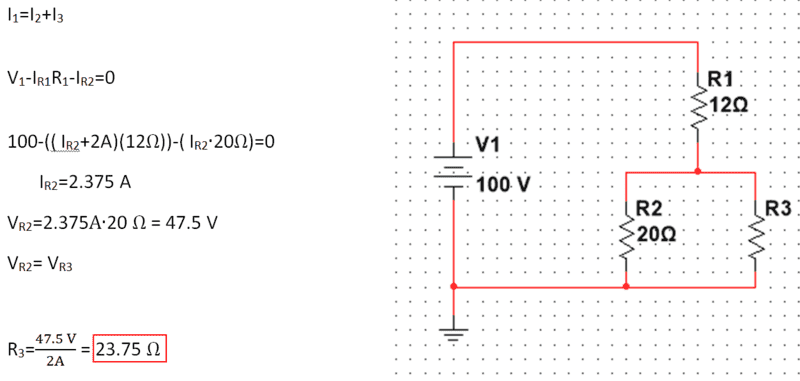Thanks again to everyone that offered their assistance!

#### Attachments

Since the OP has arrived at a correct solution though his efforts, I thought I'd present two alternative methods based on the hints that I gave earlier (Nodal analysis and Thevenin Equivalents). I understand that the OP may not have covered these techniques yet in their course, but for those who have (or who have read ahead) it might prove useful to see that there are often multiple ways to analyze a circuit and that some techniques can turn what would otherwise involve a fair bit of algebra involving simultaneous equations into something relatively straightforward.

First Method: Nodal Analysis
Consider the node equation for the node labeled ##V_x## in the following figure: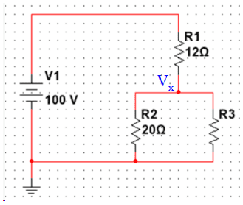Summing the currents leaving the node we have:

##\frac{V_x - 100}{12} +\frac{V_x}{20} + \frac{V_x}{R3} = 0##

Now we know that the current through ##R3## is a given 2 A, so the equation becomes:

##\frac{V_x - 100}{12} +\frac{V_x}{20} + 2 = 0##

so that

##V_x = \frac{95}{2}~V ## or 47.5 V.

We now know the potential across ##R3## as well as the current through it, so by Ohm's law:

##R3 = \frac{95/2}{2} = \frac{95}{4}~Ω = 23.75~Ω##

Second Method: Thevenin Equivalent
Taking ##R3## to be the "load" on the rest of the network, we remove that load and find the Thevenin Equivalent for the remaining source and resistor network: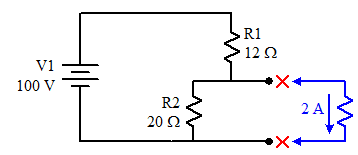##R_{th} = \frac{12⋅20}{12 + 20} = \frac{15}{2} = 7.5~Ω ##

##V_{th} = 100 \frac{20}{12 + 20} = \frac{125}{2} = 62.5~V##

So the circuit can be reduced to: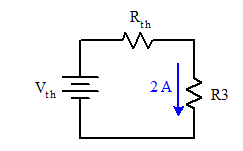Since the current in this series circuit is by inspection 2 A, we can invoke Ohm's law:

##I = \frac{V_{th}}{R_{th} + R3}~~~~## or numerically, ##~~~~2 = \frac{62.5}{7.5 + R3}##

Solving for R3:

##R3 = \frac{1}{2} 62.5 - 7.5 = 23.5~Ω##

Edit: Corrected a couple of minor typo's in the verbiage. The equations are untouched.

#### Attachments

Last edited:
•kibara